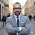# Projecting 3D points to a 2D screen coordinate system

In this article I will put forward a small function to project 3D points to a 2D coordinate system. I have used VLO framework to display all the points after the calculation of different common functions. The most difficult part is to correctly project 3D points into a 2D perspective. Basically the way to display the points is by using a viewport and setting it up correctly. Once all the points are calculated, we need to apply the transformations matrix to position the viewport and then draw the 3D object projected into a 2D canvas from the viewport perspective. The following function will use rotation matrix to correctly position the points.

Sample code:
```procedure T3DMesh.CalcPoints();
function d3Dto2D (viewport: T3DViewPort; point : T3DPoint; centre : T2DPoint; zoom : double) : T2DPoint;
var
p : T3DPoint;
t : T3DPoint;
d2d : T2DPoint;
begin
p.x := viewport.x + point.x;
p.y := viewport.y + point.y;
p.z := viewport.z + point.z;

t.x := (x * cos(viewport.roll)) - (z * sin(viewport.roll));
t.z := (x * sin(viewport.roll)) + (z * cos(viewport.roll));
t.y := (y * cos(viewport.pitch)) - (t.z * sin(viewport.pitch));
z := (t.y * cos(viewport.pitch)) - (t.z * sin(viewport.pitch));
x := (t.x * cos(viewport.yaw)) - (t.y * sin(viewport.yaw));
y := (t.x * sin(viewport.yaw)) + (t.y * cos(viewport.yaw));
d2d := nil;
if z > 0 then
begin
d2d := T2DPoint.Create(((x / z) * zoom) + centre.x, ((y / z) * zoom) + centre.y);
end;
result := d2d;
end ;

var
listPoint : TObjectList;
i: Integer;
j: Integer;
x, y, z: Double;
d2dpoint : T2DPoint;
begin
listPoint := TObjectList.Create;
listPoint2 := TObjectList.Create;

x :=-2.0;
y := -2.0;
z := 0.0;
for i := 0 to 40 do
begin
y := -2.0;
for j := 0 to 40 do
begin
z := cos((x * x + y * y) * 2) * exp(-1 * ((x * x) + (y * y)));
y := y + 0.1;
end;
x := x + 0.1;
end;

for i := 0 to listPoint.count - 1 do
begin
d2dpoint := d3Dto2D(viewport, T3DPoint(listPoint[i]), centre, zoom);
if Assigned(d2dpoint) then
end;
listPoint.Free;
end;
```

Examples:
z = sin(x) * cos (y):

z = cos((x^2+y^2)*2) * exp-(((x^2)+(y^2))):
1.1.